# AngularJS Expressions: ARRAY, Objects, \$eval, Strings [Examples]

### What is Angular JS Expressions?

Expressions are variables which were defined in the double braces {{ }}. They are very commonly used within Angular JS, and you would see them in our previous tutorials.

In this tutorial, you will learn-

## Explain Angular.js Expressions with an example

AngularJS expressions are those that are written inside double braces {{expression}}.

Syntax:

A simple example of an expression is {{5 + 6}}.

Angular.JS expressions are used to bind data to HTML the same way as the ng-bind directive. AngularJS displays the data exactly at the place where the expression is placed.

Let’s look at an example of Angular.JS expressions.

In this example, we just want to show a simple addition of numbers as an expression.

```<html>
<meta chrset="UTF 8">
<title>Event Registration</title>

<body>

<script src="https://code.angularjs.org/1.6.9/angular-route.js"></script>
<script src="https://code.angularjs.org/1.6.9/angular.min.js"></script>

<h1> Guru99 Global Event</h1>

<div ng-app="">
</div>

</body>
</html>```

Code Explanation:

1. The ng-app directive in our example is blank as shown in above screenshot. This only means that there is no module to assign controllers, directives, services attached to the code.
2. We are adding a simple expression which looks at the addition of 2 numbers.

If the code is executed successfully, the following Output will be shown when you run your code in the browser.

Output: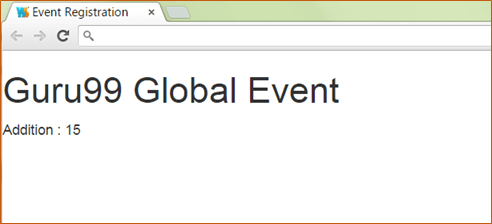From the output,

• It can be seen that the addition of the two numbers 9 and 6 take place and the added value of 15 is displayed.

## Angular.JS Numbers

Expressions can be used to work with numbers as well. Let’s look at an example of Angular.JS expressions with numbers.

In this example, we just want to show a simple multiplication of 2 number variables called margin and profit and displayed their multiplied value.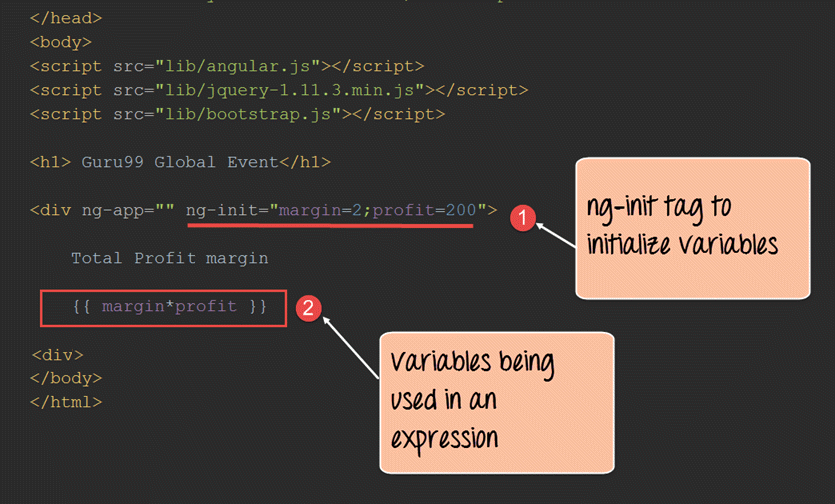```<!DOCTYPE html>
<html>
<meta chrset="UTF 8">
<title>Event Registration</title>

<body>

<script src="https://code.angularjs.org/1.6.9/angular-route.js"></script>
<script src="https://code.angularjs.org/1.6.9/angular.min.js"></script>

<h1> Guru99 Global Event</h1>

<div ng-app="" ng-init="margin=2;profit=200">
Total profit margin

{{margin*profit}}
</div>

</body>
</html>```

Code Explanation:

1. The ng-init directive is used in angular.js to define variables and their corresponding values in the view itself. It’s somewhat like defining local variables to code in any programming language. In this case, we are defining 2 variables called margin and profit and assigning values to them.
2. We are then using the 2 local variables and multiplying their values.

If the code is executed successfully, the following Output will be shown when you run your code in the browser.

Output: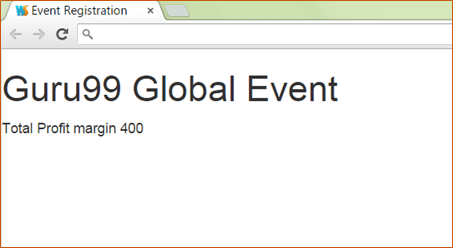From the output,

• It can be clearly seen that the multiplication of the 2 numbers 2 and 200 take place, and the multiplied value of 400 is displayed.

## AngularJS Strings

Expressions can be used to work with strings as well. Let’s look at an example of Angular JS expressions with strings.

In this example, we are going to define 2 strings of “firstName” and “lastName” and display them using expressions accordingly.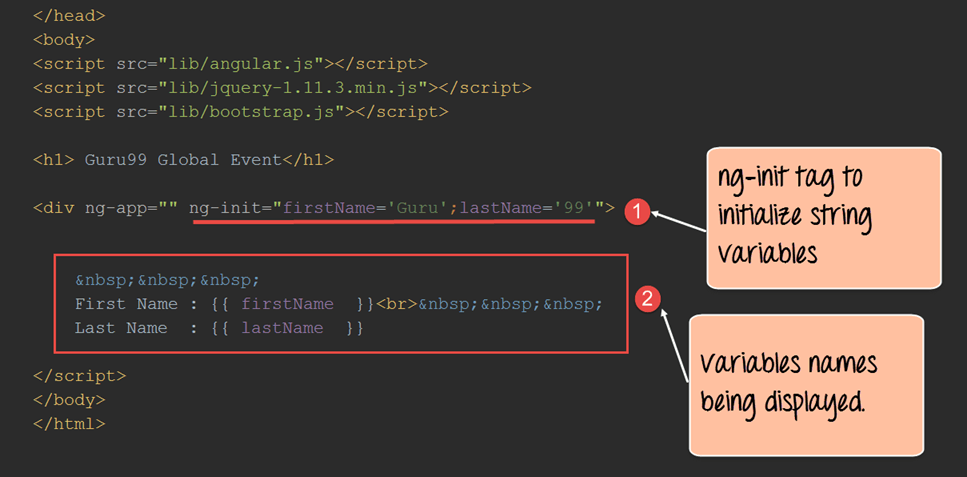```<!DOCTYPE html>
<html>
<meta chrset="UTF 8">
<title>Event Registration</title>

<body>

<script src="https://code.angularjs.org/1.6.9/angular-route.js"></script>
<script src="https://code.angularjs.org/1.6.9/angular.min.js"></script>

<h1> Guru99 Global Event</h1>

<div ng-app="" ng-init="firstName='Guru';lastName='99'">

&nbsp;&nbsp;&nbsp;
First Name : {{firstName}}<br>&nbsp;&nbsp;&nbsp;
last Name : {{lastName}}

</div>

</body>
</html>```

Code Explanation:

1. The ng-init directive is used define the variables firstName with the value “Guru” and the variable lastName with the value of “99”.
2. We are then using expressions of {{firstName}} and {{lastName}} to access the value of these variables and display them in the view accordingly.

If the code is executed successfully, the following Output will be shown when you run your code in the browser.

Output: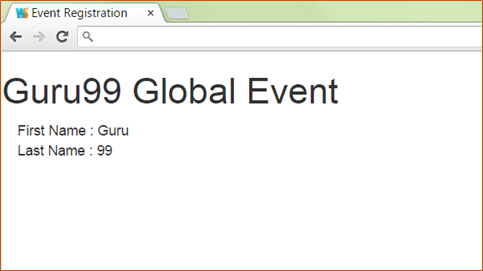From the output, it can be clearly seen that the values of firstName and lastName are displayed on the screen.

## Angular.JS Objects

Expressions can be used to work with JavaScript objects as well.

Let’s look at an example of Angular.JS expressions with javascript objects. A javascript object consists of a name-value pair.

Below is an example of the syntax of a javascript object.

Syntax:

`var car = {type:"Ford", model:"Explorer", color:"White"};`

In this example, we are going to define one object as a person object which will have 2 key value pairs of “firstName” and “lastName”.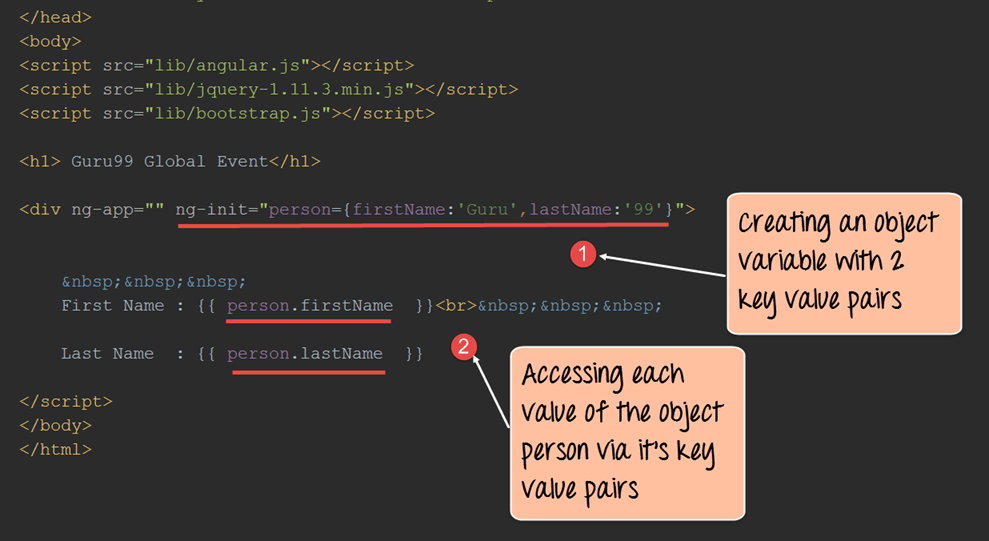```<!DOCTYPE html>
<html>
<meta chrset="UTF 8">
<title>Event Registration</title>

<body>

<script src="https://code.angularjs.org/1.6.9/angular-route.js"></script>
<script src="https://code.angularjs.org/1.6.9/angular.min.js"></script>

<h1> Guru99 Global Event</h1>

<div ng-app="" ng-init="person={firstName:'Guru',lastName:'99'}">

&nbsp;&nbsp;&nbsp;
First Name : {{person.firstName}}<br>&nbsp;&nbsp;&nbsp;
Last Name : {{person.lastName}}

</div>

</body>
</html>```

Code Explanation:

1. The ng-init directive is used to define the object person which in turn has key value pairs of firstName with the value “Guru” and the variable lastName with the value of “99”.
2. We are then using expressions of {{person.firstName}} and {{person.secondName}} to access the value of these variables and display them in the view accordingly. Since the actual member variables are part of the object person, they have to access it with the dot (.) notation to access their actual value.

If the code is executed successfully, the following Output will be shown when you run your code in the browser.

Output:From the output,

• It can be clearly seen that the values of “firstName” and “secondName” are displayed on the screen.

## AngularJS Arrays

Expressions can be used to work with arrays as well. Let’s look at an example of Angular JS expressions with arrays.

In this example, we are going to define an array which is going to hold the marks of a student in 3 subjects. In the view, we will display the value of these marks accordingly.```<html>
<meta chrset="UTF 8">
<title>Event Registration</title>
<body>

<script src="https://code.angularjs.org/1.6.9/angular-route.js"></script>
<script src="https://code.angularjs.org/1.6.9/angular.min.js"></script>

<h1> Guru99 Global Event</h1>

<div ng-app="" ng-init="marks=[1,15,19]">

Student Marks<br>&nbsp;&nbsp;&nbsp;
Subject1 : {{marks }}<br>&nbsp;&nbsp;&nbsp;
Subject2 : {{marks }}<br>&nbsp;&nbsp;&nbsp;
Subject3 : {{marks }}<br>&nbsp;&nbsp;&nbsp;
</div>

</body>
</html>```

Code Explanation:

1. The ng-init directive is used define the array with the name “marks” with 3 values of 1, 15 and 19.
2. We are then using expressions of marks [index] to access each element of the array.

If the code is executed successfully, the following Output will be shown when you run your code in the browser.

Output:From the output, it can be clearly seen that the marks being displayed, that are defined in the array.

## AngularJS Expression capabilities and Limitations

Angular.JS Expression capabilities

1. Angular expressions are like JavaScript expressions. Hence, it has the same power and flexibility.
2. In JavaScript, when you try to evaluate undefined properties, it generates a ReferenceError or TypeError. In Angular, expression evaluation is forgiving and generate an undefined or null.
3. One can use filters within expressions to format data before displaying it.

Angular JS Expression limitations

1. There is currently no availability to use conditionals, loops, or exceptions in an Angular expression
2. You cannot declare functions in an Angular expression, even inside ng-init directive.
3. One cannot create regular expressions in an Angular expression. A regular expression is a combination of symbols and characters, which are used to find for strings such as .*\.txt\$. Such expressions cannot be used within Angular JS expressions.
4. Also, one cannot use, or void in an Angular expression.

## Difference between expression and \$eval

The \$eval function allows one to evaluate expressions from within the controller itself. So while expressions are used for evaluation in the view, the \$eval is used in the controller function.

Let’s look at a simple example on this.

In this example,

We are just going to use the \$eval function to add 2 numbers and make it available in the scope object so that it can be shown in the view.```<!DOCTYPE html>
<html>
<meta chrset="UTF 8">
<title>Event Registration</title>
<body>

<script src="https://code.angularjs.org/1.6.9/angular-route.js"></script>
<script src="https://code.angularjs.org/1.6.9/angular.min.js"></script>
<script src="https://code.jquery.com/jquery-3.3.1.min.js"></script>

<div ng-app="sampleApp" ng-controller="AngularController">
<h1> Guru99 Global Event</h1>
{{value}}
</div>
<script>
var sampleApp = angular.module('sampleApp',[]);
sampleApp.controller('AngularController',function(\$scope){
\$scope.a=1;
\$scope.b=1;

\$scope.value=\$scope.\$eval('a+b');
});
</script>

</body>
</html>```

Code Explanation:

1. We are first defining 2 variables ‘a’ and ‘b’, each holding a value of 1.
2. We are using the \$scope.\$eval function to evaluate the addition of the 2 variables and assigning it to the scope variable ‘value’.
3. We are then just displaying the value of the variable ‘value’ in the view.

If the code is executed successfully, the following Output will be shown when you run your code in the browser.

Output:The above output shows the output of the expression which was evaluated in the controller. The above results show that the \$eval expression was used to perform the addition of the 2 scope variables ‘a and b’ with the result sent and displayed in the view.

Summary:

• We have seen how expressions in Angular JS can be used to evaluate regular JavaScript like expressions such as the simple addition of numbers.
• The ng-init directive can be used to define variables in-line which can be used in the view.
• Expressions can be made to work with primitive types such as strings and numbers.
• Expressions can also be used to work with other types such as JavaScript objects and arrays.
• Expressions in Angular JS does have a few limitations like for example not being to have regular expressions or use functions, loops or conditional statements.